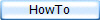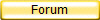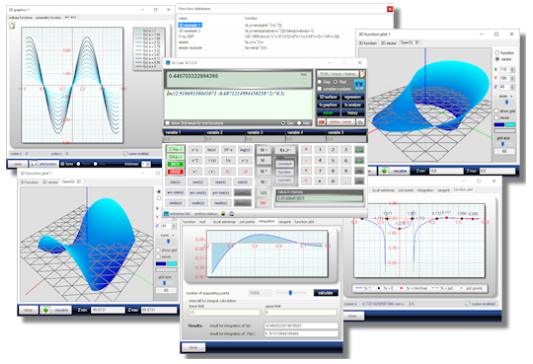new video tutorials ...

fx-Calc
is actually one of the most advanced desktop calculators for Windows.
The biggest advantage is the simple and intuitive handling of complex mathematical tasks.
Starting with a familiar calculator window, it allows calculating self defined functions, sums and products. It takes for instance just a few clicks to run the Leibnitz iteration to calculate Pi.

fx-Calc lets you define, analyse, visualise and calculate scientific functions and is a full featured replacement and/or enhancement of the old fashioned Windows calculator. In addition to calculation and visualization you can analyze and solve scientific functions and perform linear regression.
Even if the Window concept may not look advanced, it provides the big advantage of opening similar windows multiple times to compare the results as needed.

To use the interpreter DLL in your own projects, you can obtain a copy and documentation of this DLL directly from the author.

### Key features:

• definition and immediate calculation of functions with up to 5 variables
• solves equations
• function analysis combined with graphic result presentation
• roots, extrem - and pole points , integration
• tangent calculation and interactive Newton iteration
• parametric sum and product calculation
• integrated function library
• integrated library for scientific constants
• 2D function plots including complex graphs
• 2D prametric function plots
• OpenGL accelerated 3D function plots
• multiple plot and analysis windows to compare different function characteristics
• interactive linear regression module
• assistant for combinatorics
• automated history
• displays current memory value in separate field
• integrated examples
• is handling complex numbers
• syntax highlightingWindows Installer XP/Vista/Win7/Win8/Windows 10 32 & 64 bit version (pay attention to dependencies !) fxcsetup_en.exe Version 4.9.3.2 07.07.2020 1.7 MByte### Dependencies:

• Microsoft .Net framework version 4 and above